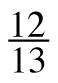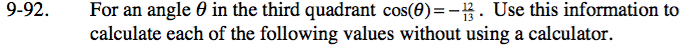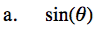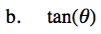### Home > INT3 > Chapter 9 > Lesson 9.1.7 > Problem9-92

9-92.
1. For an angle θ in the third quadrant cos(θ) = –. Use this information to calculate each of the following values without using a calculator. Homework Help ✎

1. sin(θ)

2. tan(θ)Draw a unit circle and label the triangle for the given angle.